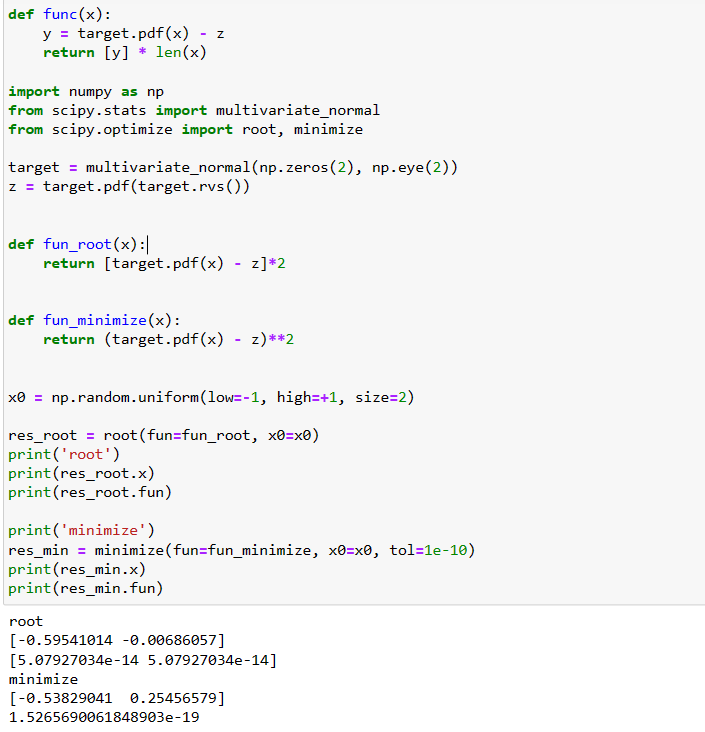# Finding the roots of a function using SciPy's root functionby kanikaUpdated: Mar 29, 2023Solution Kit

SciPy's root function can be used to find the roots of a function. To do this, you need to define the function and its derivatives. Then, you can use the root function to find the roots of the function by passing it a set of starting points. The root function will then use an iterative algorithm to find the roots of the function.

• SciPy: Scipy is a free, open-source Python library for scientific and technical computing. It contains modules for optimization, linear algebra, integration, interpolation, special functions, FFT, signal, and image processing.

• SciPy root function: The SciPy root function is a numerical root-finding function in the SciPy library. It calculates the root of a given equation, i.e., the variable's value that makes the equation equal to zero. This function can be used for both univariate and multivariate equations.

Here is an example of finding the roots of a function using SciPy's root function.Fig 1: Preview of the output that you will get on running this code from your IDE

### Code

In this solution, we use the root Function of the SciPy library.

### Instructions

Follow the steps carefully to get the output easily.

1. Install Jupyter Notebook on your computer.
2. Open terminal and install the required libraries with following commands.
3. Install numpy - pip install numpy.
4. Install scipy - pip install scipy.
5. Copy the code using the "Copy" button above, and paste it into your IDE's Python file.
6. Run the file to find the roots of function using SciPy's root function.

I hope you found this useful. I have added the link to dependent libraries, version information in the following sections.

I found this code snippet by searching for "Finding the root of function using SciPy's root function" in kandi. You can try any such use case!

### Dependent Libraries

numpyby numpy

Python23755Version:v1.25.0rc1The fundamental package for scientific computing with Python.

Support
Quality
Security
Reuse

numpyby numpy

Python23755Version:v1.25.0rc1License: Permissive (BSD-3-Clause)

The fundamental package for scientific computing with Python.
Support
Quality
Security
Reuse

scipyby scipy

Python11340Version:v1.11.0rc1SciPy library main repository

Support
Quality
Security
Reuse

scipyby scipy

Python11340Version:v1.11.0rc1License: Permissive (BSD-3-Clause)

SciPy library main repository
Support
Quality
Security
Reuse

If you do not have SciPy or numpy that is required to run this code, you can install it by clicking on the above link and copying the pip Install command from the numpy page in kandi.

You can search for any dependent library on kandi like numpy

### Environment Tested

I tested this solution in the following versions. Be mindful of changes when working with other versions.

1. The solution is created in Python 3.9.6
2. The solution is tested on SciPy version 1.9.3

Using this solution, we are able to find roots of function using SciPy's root function. It can also be used for optimization, numerical integration, and interpolation.

See similar Kits and Libraries